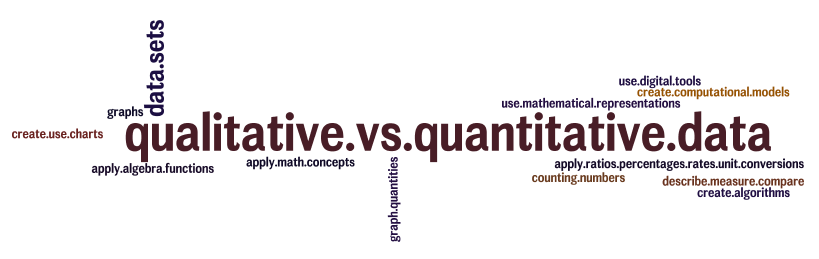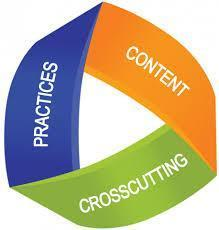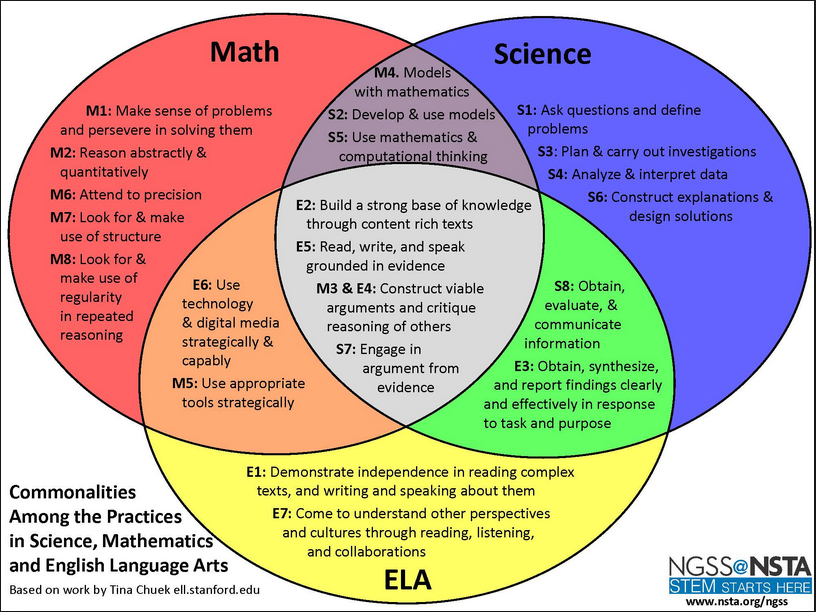Using Mathematics & Computational Thinking Toolkit Overview Video for this Practice BUILDING AWARENESSFramework Rationale Science In science, mathematics and computation are fundamental tools for representing physical variables and their relationships. They are used for a range of tasks, such as constructing simulations, statistically analyzing data, and recognizing, expressing, and applying quantitative relationships. Mathematical and computational approaches enable predictions of the behavior of physical systems, along with the testing of such predictions. Moreover, statistical techniques are invaluable for assessing the significance of patterns or correlations.Engineering In engineering, mathematical and computational representations of established relationships and principles are an integral part of design. For example, structural engineers create mathematically based analyses of designs to calculate whether they can stand up to the expected stresses of use and if they can be completed within acceptable budgets. Moreover, simulations of designs provide an effective test bed for the development of designs and their improvement.See A Framework for K-12 Science Education, 2011, p. 64 for entire text. BUILDING AWARENESS QUESTIONS 1.  From the background information, what new awareness do you have about mathematics and computational thinking? 2.  In a 3-Dimensional classroom, who do you think mathematics and computational thinking applies?3.  What questions did the background raise for you? 4.  Upack this Practice by identifying the verbs and nouns in the description.  Is your list similar to this?EXPOSING BELIEFS Using Mathematics & Computational Thinking podcastEXPOSING BELIEFQUESTIONS 1.  What are your current beliefs about this practice? 2.  In what ways do you think you are using this practice? 3.  What challenges do you see to using this practice? CONFRONTING BELIEFS Conceptual Change Activities: Using Mathematics #1: A Helping of Pi Using Mathematics #2: Pendulums are mathematically beautiful Using Computational Thinking #1:  Pendulum simulation Using Computational Thinking #2:  Wind Power

Developing Conceptual Understanding of Using Mathematics and Computational Thinking

The purpose of the following activities is to engage learners in the Practice of Using Mathematics and Computational Thinking.  The emphasis is NOT on the activity itself, but rather the conceptual change related to the practice.  Consumers of the Toolkit are reminded to not get wrapped up in the activity, but rather continually reflect on the conceptual nature of the practice to gain deeper understanding.

Since the following activities are NOT lesson plans, in some cases only a brief explanation of the activity has been provided.  The facilitator should encourage the learners to direct their own investigations and only intervene as needed to redirect.

Using Mathematics #1:  A Helping of Pi

Learners can discover Pi for themselves using this activity.

Materials:

A variety of sizes of 5 or 6 hoola hoops
String

Meter sticks

Vocabulary preview:

C = Circumference- the measurement of the outside of a circle
R = radius- the measurement of a circle from the center point to a point on the
circle
D = diameter – the measurement of a circle from one point on the circle, through
the center point, to the point on the circle on the opposite side.

Pi = 3.14 the number that is used in the formula to find the circumference of a circle determined based on how many degrees are in a circle.

Inquiry Questions:

1. Is there a way to calculate the circumference of a circle without actually measuring it?

2. Why might you want to know the circumference of a circle?

Everyday life applications:

How Scientists and Engineers might use this information:

Activity:

1. Before learners come in the classroom, draw a large chalk circle in the middle of the room using a 4 to 5 foot piece of string attached to a central pivot point. (the leg of a chair or desk)

2. Tell learners that they need to build a: Leprechaun pen, sheep pen, any number of suggested round enclosures: Your task is to find out how much fencing material you will need for your enclosure.

3. Ask the learners to brainstorm a variety of ways that they might determine the amount of material they will need to build their enclosure. Have them work in pairs and write their ideas in their notebooks.

4. Explain how scientists and mathematicians use proven formulas as tools to solve problems.

5. Tell learners how you drew the large circle in the room. Ask, “If I attached the string to a fixed point in the center of the circle, would that be considered the diameter or radius? If we know the radius, this is the formula we would use to determine the circumference: Write this formula on the board: C=r2π

Point to each symbol or letter to ensure that learners understand what each stands for.

6. We know what the radius is (tell them what length of string you used) can we calculate the circumference? Hopefully, they will see that they have all of information they need in order to perform the calculations. Let them work on solving the problem, then do the calculations again to check students’ work.

7. Now, break the class into groups of 4 and give each group a hoola hoop, a meter stick, and string. Tell them that they won’t be able to calculate the radius accurately without know the exact center point, so what might you do with the tools that you have to determine the circumference of your hoola hoop, using what you know about radius and diameter. Consider the formula and look back at definitions of each vocabulary word.

8. Let them think for a while, then check to see if any groups discovered the formula C=dπ. If they didn’t, guide them to this step and have them determine the circumference of their hoops.

9. Last, have them use their string as a measurement device, laying their string around the outside of the hoop. Now they will measure their strings to see if their string length matches what their formula said that the circumference would be.

10. List each group’s measurements on the board. Discuss, argue about, and justify whether each group’s calculations were reasonable and why or why not.

11. Go back to the application ideas that your students wrote in their notebooks. Ask them if they had any other ideas as they were doing this activity for science and engineering.

Technology:

Research what mathematician developed Pi and the formula for circumference.
Go to the illustrative math website for MCTM,   Search “circles” and click on

illustrations. They will be able to manipulate a circle of their own design and see what happens with the circumference.

Using Mathematics #2:  Pendulums are Mathematically Beautiful

After learners have been introduced to pendulums...see Planning and Carrying Out Investigations Activity #2 and Analyzing and Interpreting Data Activity #1...they can begin to examine the mathematical properties of pendulums.

Take an existing data table of pendulum length and frequency.  Discuss with learners the mathematical methods that can be used with numbers...division, multiplication, etc.  Eventually arrive at the notion of squaring a number.  Have learners create a third column in their data table and square the frequency.  Next, graph the squared frequency against the length, keeping in mind that length is the independent variable and it is the X axis.

See Square It, page 9, TOP Learning Systems Pendulums 01

Using Computational Thinking #1:  Pendulum simulation

Engage learners in computer simulations.  The following are pendulum simulations:

Simple Pendulum  Requires Java Applet

Double Pendulum  Requires Java Applet

Using Computational Thinking #2:  Wind Power

Introduce learners to wind power using websites such as the following:

National Geographic Wind Power
Wind Turbine Simulator

Learners can explore background information about wind generated power and use the online simulations to create multiple scenarios of variables that affect wind power.  Learners should be encouraged to continually ask questions, record findings, and engage in argumentation when sharing results.

 REINTERPRETING BELIEFS Debrief the activity(ies) by focusing on the conceptual understanding of the practice using the following prompts: REINTERPRETING BELIEFS QUESTIONS 1.  In what ways did this activity change your beliefs about using mathematics and computational thinking? 2.  How difficult was it to use mathematics and computational thinking? 3.  What clarity was brought to the use of mathematics and computational thinking through these activities? INTEGRATION QUESTIONS 1.  How do you currently help students to use mathematics and computational thinking in your classroom? 2.  Review a recent lesson you taught and evaluate the effectiveness of using mathematics and computational thinking. INVENTION QUESTIONS 1. Share lessons in which you could implement the practice of using mathematics and computational thinking. 2. Ask a colleague to observe one of your lessons OR video yourself teaching and reflect specifically on using mathematics and computational thinking. 3. Use the EQuiP Rubric for Lessons & Units:  Science to evaluate a recent science lesson you taught.

Learning Progression for Mathematics and Computational Thinking

Elementary:  Students should be encouraged to use mathematics and computational thinking in ALL areas of science.  This begins with an awareness of mathematics in science.  Students should learn to recognize and describe number patterns and use appropriate instruments such as rulers, protractors, thermometers and more.

Middle School:  Students continue to use mathematics and computational thinking, but need to see relationships expressed in both words and algebraic symbols.  The use of computers and other technologies to aid in mathematics and computational thinking is necessary

High School:  Students should be more proficient at mathematics and computational thinking, exploring ways to use computers for data analysis, simulations and more.

See p. 10  Appendix F Science and Engineering Practices in the NGSS for a more thorough grade band progression.

Connection to Common Core State Standards in mathematics and English language arts.Conceptual Change and NGSS

Asking Questions in Science & Defining Problems in Engineering

Developing and Using Models

Planning & Carrying Out Investigations

Analyzing & Interpreting Data

Using Mathematics & Computational Thinking

Constructing Explanations & Designing Solutions

Engaging in Argument

Obtaining, Evaluating & Communicating